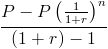# Present Value of Annuity

PV of Annuity Calculator (Click Here or Scroll Down)The present value of annuity formula determines the value of a series of future periodic payments at a given time. The present value of annuity formula relies on the concept of time value of money, in that one dollar present day is worth more than that same dollar at a future date.

## Rate Per Period

As with any financial formula that involves a rate, it is important to make sure that the rate is consistent with the other variables in the formula. If the payment is per month, then the rate needs to be per month, and similarly, the rate would need to be the annual rate if the payment is annual.

An example would be an annuity that has a 12% annual rate and payments are made monthly. The monthly rate of 1% would need to be used in the formula.

## Assumptions

The formula shown has assumptions, in that it must be an ordinary annuity. These assumptions are that

1) The periodic payment does not change
2) The rate does not change
3) The first payment is one period away

If the payment and/or rate changes, the calculation of the present value would need to be adjusted depending on the specifics. If the payment increases at a specific rate, the present value of a growing annuity formula would be used.

If the first payment is not one period away, as the 3rd assumption requires, the present value of annuity due or present value of deferred annuity may be used. An annuity due is an annuity that's initial payment is at the beginning of the annuity as opposed to one period away. A deferred annuity pays the initial payment at a later time.

## How is the PV of Annuity Formula derived?

The present value of a series of payments, whether the payments are the same or not, isWhen the periodic payments or dividends are all the same, this is considered a geometric series. By using the geometric series formula, the formula can be rewritten asThis equation can be simplified by multiplying it by (1+r)/(1+r), which is to multiply it by 1. Notice that (1+r) is canceled out throughout the equation by doing this. The formula is now reduced toThe P's in the numerator can be factored out of the fraction and become 1. The 1's in the denominator of the formula are subtracted from one another. After making these adjustments, the formula is simplified to the present value of annuity formula shown on the top of the page.

New to Finance?